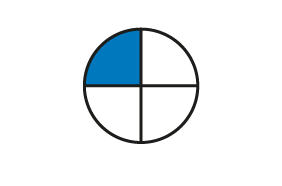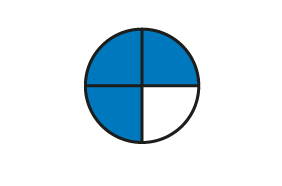Home > Fractions > Big ideas > Fractions as operators > Using the operator model

# Using the operator model

When students begin working with fractions, their thinking and modelling is based on part-whole thinking.

Initially, tasks tend to focus on unit fractions modelled first with area (shapes) and then with discrete materials (groups of items).

For example:

 Show $$\frac{1}{4}$$ of the circle. What is $$\frac{1}{4}$$ of 8?Area model of unit fractions.Discrete model of unit fractions.

When tasks move to non-unit fractions, student thinking is likely to be additive, as they begin with one part and build up to the required number of parts.

 Show $$\frac{3}{4}$$ of the circle. What is $$\frac{3}{4}$$ of 8?Area model of non-unit fractions.Discrete model of non-unit fractions.

To effectively use fractions as operators, student thinking needs to be multiplicative rather than additive. For example, to find $$\frac{3}{4}$$ of 8, divide by 4, then multiply by 3.

As shown in the operator animation, first modelling the process with arrays of items, then moving into grids and area diagrams can help achieve the required multiplicative thinking. Watch the video Fractions as Operators: Modelling the Operators.

The area model is particularly useful for modelling the operation of a fraction on another fraction: as in $$\frac{3}{4} \times \frac{3}{4}$$.